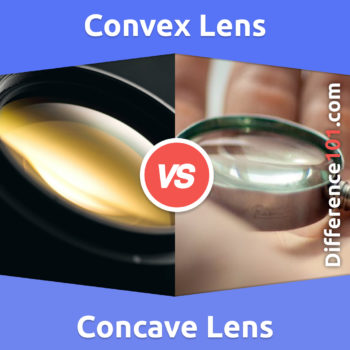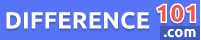# Convex vs. Concave Lens: What Is the Difference Between Convex and Concave Lens?

Do you know the difference between concave and convex lenses? How they work? The different shapes of these lenses and how they are used are discussed in this article.Convex vs. Concave Lens: The lens allows us to see things, different colors, and distinguish between different objects. Our eyes have a crystalline lens through which we see the whole world. Lenses have different curves and shapes. Lenses are divided into two types on the basis of shapes: convex and concave lenses. Sometimes both lenses are combined together to give a clearer image. But in concave vs. Convex lens, the concave lens disperse the light rays while the convex lens merges the light rays at a particular point.

Let’s take a closer look at Convex versus Concave Lenses

## What are Convex Lens?A convex lens is a lens that has thin edges but is thicker at the center. This lens can convert the light rays into beams. A convex lens can make both virtual and real images depending upon the position of the object. The convex lens is also used to start a fire with the rays of the sun, and most children have tried this after studying physics. This lens is used for hypermetropia. There are many other uses of the lens as well, like making optical instruments, microscopes, etc.

👉  Glock 17 vs. 19: What is the difference between Glock 17 and 19?

## What are Concave Lens?A concave lens is the type of lens that is thinner from the center but has thicker edges. This lens has the ability to spread the light rays, also known as a diverging lens. The image formed by the concave lens is always smaller than the object. The image is also virtual and upright. The size of the image also depends upon the distance of the object from the lens. Concave lenses are used in telescopes, side mirrors, movie projectors, etc.

## Convex Lens Examples

A few examples of the convex lens are the human eye, eyeglasses, microscope, projectors, cameras, telescope, projectors.

👉  What Is The Difference Between 4G and LTE?

## Concave Lens Examples

There are a few examples of concave lenses: binoculars, telescopes, eyeglasses to correct short-sightedness, flashlight, and laser-like CD and DVD player.

## Convex vs. Concave Lens Similarities

• Both the convex and concave lenses are made up of glass or transparent material.
• Both lenses have at least one curved surface.
• Both lenses, convex and concave, have a smooth surface.

## Pros and Cons of a Convex Lens### Pros of a Convex Lens

•  A convex lens is used to magnify the objects, as it can make the object look bigger and larger.
• Convex lenses are also used in cameras to focus the image, but moving the lens, the magnification of the image can be changed.
• Peepholes are small holes on the house’s front door for security purposes. These peepholes also have a convex lens to clearly see who is outside.

### Cons of a Convex Lens

• Convex lenses, when used in binoculars and telescopes for magnifying, do not transfer light accurately and can cause distortion and blur.
• The convex lens in the camera refracts the light of different colors and creates the fringe effect on the bright objects present in the picture.

## Pros and cons of a Concave Lens### Pros of a Concave Lens

• The concave lens used in the flashlight can increase the light source’s radius and give w wider beam
• Photographers used concave lenses with convex lenses in their cameras to improve the quality of the images.

### Cons of a Concave Lens

• The concave lens can make the objects look smaller and shorter due to the thinner center.
• A Concave lens forms both inverted and real images; depending upon the object’s position, it can form either a magnified or diminished image.

## Comparison Chart## FAQs

### Is a Converging Lens Concave or Convex?

A convex lens is a converging lens as this lens converges the rays at one point.

👉  Wet Lab vs. Dry Lab: What Is the Difference Between Wet Lab and Dry Lab?

### What Are Convex Lenses Used For?

A convex lens is used in eyeglasses to correct far-sightedness. It is also used in cameras, microscopes, and magnifying glasses.

### Which Lens Is Used in the Telescope?

Telescope used a convex glass lens to bend the light and increase focus.

### What Is the Difference Between Concave and Convex Mirrors?

A concave mirror is a spherical mirror with an inward curve, while a convex mirror is a spherical mirror with an outward curve.

## Conclusion

Concave and convex lenses are used for clear and sharper images. Sometimes, both of these lenses are used together to get better results and are called concave-convex lenses. Both lenses are also used in many experiments, eyeglasses, telescopes, and many other things—the main difference between the convex and concave lens is due to their shape. A convex lens has a wider center and is curved inward. At the same time, the concave lens has a thinner center and is curved outward.

We will be happy to hear your thoughtsLime vs. Lemon: What’s the difference?